# MEASURING MATTER Measuring Mass A scale is used

• Slides: 24MEASURING MATTER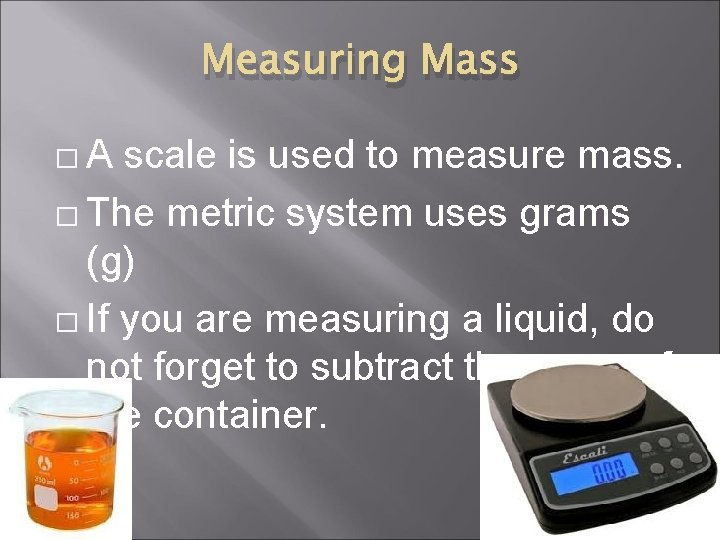Measuring Mass �A scale is used to measure mass. � The metric system uses grams (g) � If you are measuring a liquid, do not forget to subtract the mass of the container.Measuring Volume is the amount of space that an object takes up. � Which has more volume; a popcorn kernel or a piece of popped corn? �Volume of a Regular Shaped Solid � � � A regular shaped solid would be one that you could easily measure the length, width, and height using a ruler. LENGTH X WIDTH X HEIGHT Measures in cm cubed.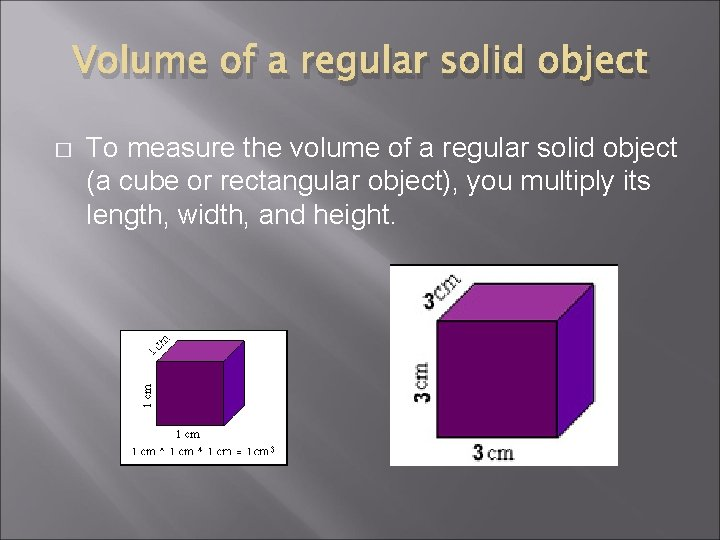Volume of a regular solid object � To measure the volume of a regular solid object (a cube or rectangular object), you multiply its length, width, and height.Volume of an Irregular Shaped Solid � � � An irregular shaped solid would be a solid object that could not be easily measured by a rule. Example: a rock or a cap eraser. When this is the case, you look at water displacement in a graduated cylinder. This is measured in ml.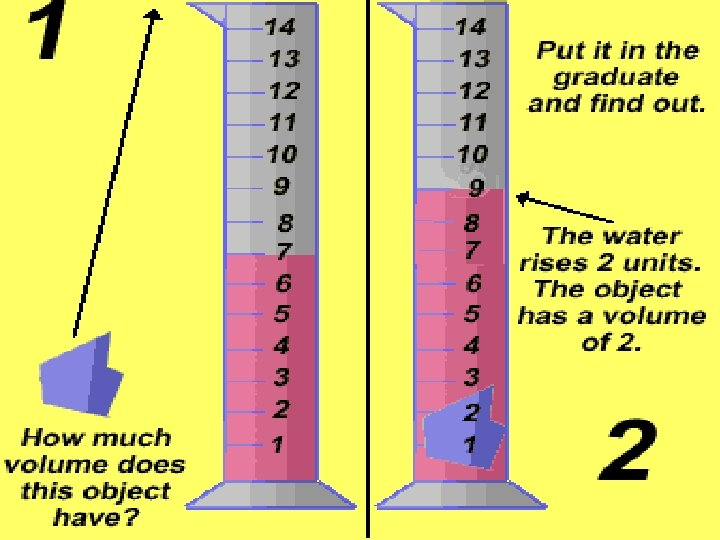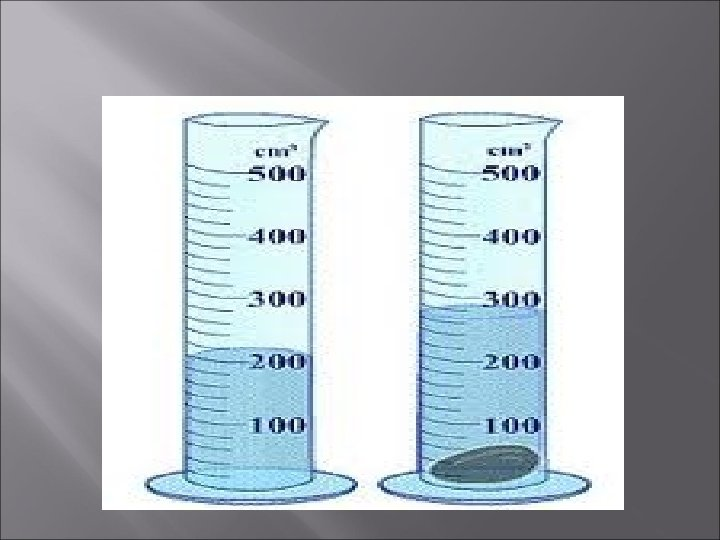Volume of a liquid � To find the volume of a liquid, you simply pour it into a beaker or a graduated cylinder and read it in ml.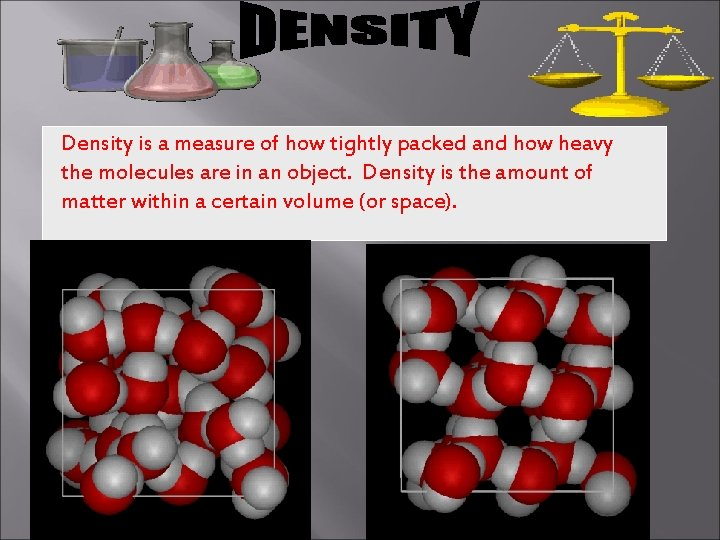Density is a measure of how tightly packed and how heavy the molecules are in an object. Density is the amount of matter within a certain volume (or space).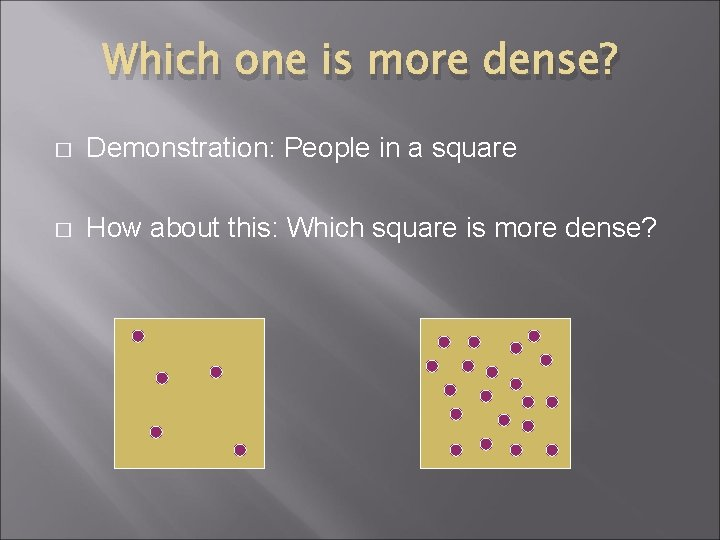Which one is more dense? � Demonstration: People in a square � How about this: Which square is more dense?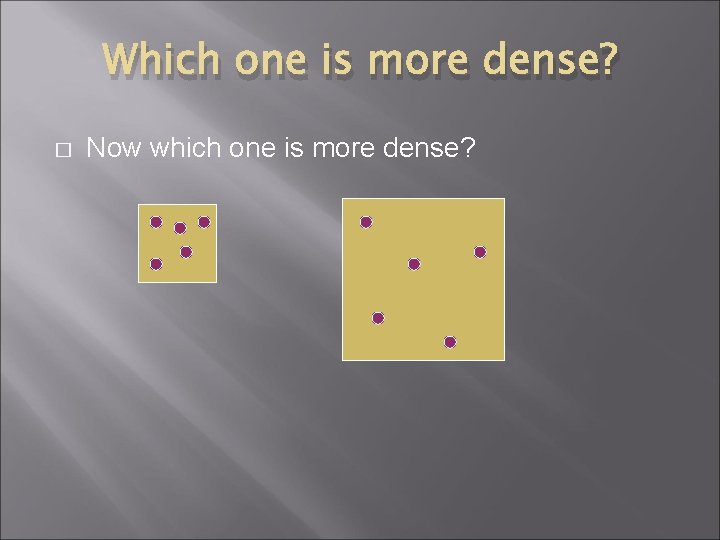Which one is more dense? � Now which one is more dense?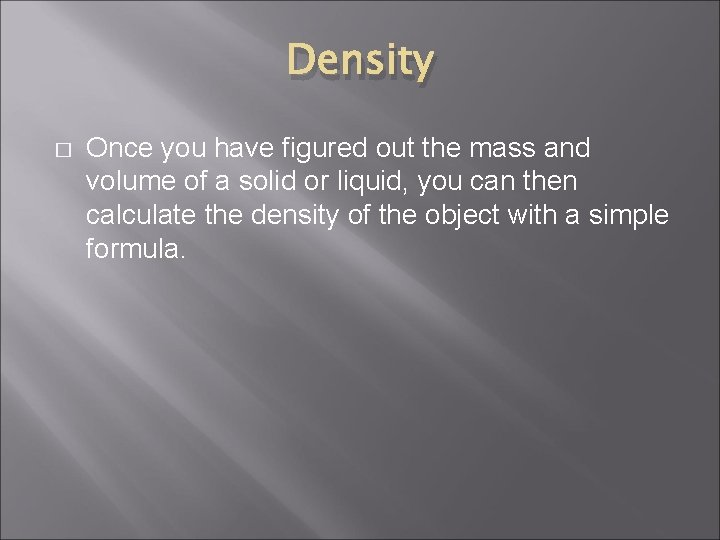Density � Once you have figured out the mass and volume of a solid or liquid, you can then calculate the density of the object with a simple formula.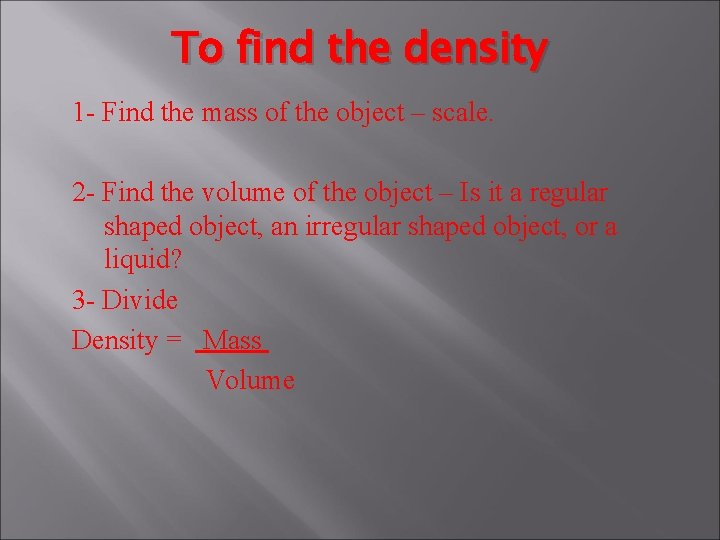To find the density 1 - Find the mass of the object – scale. 2 - Find the volume of the object – Is it a regular shaped object, an irregular shaped object, or a liquid? 3 - Divide Density = Mass VolumeUnits for density g/cm 3 or g/ml Formula: M = mass M=Dx. V V= volume D = density V=M/D D=M/V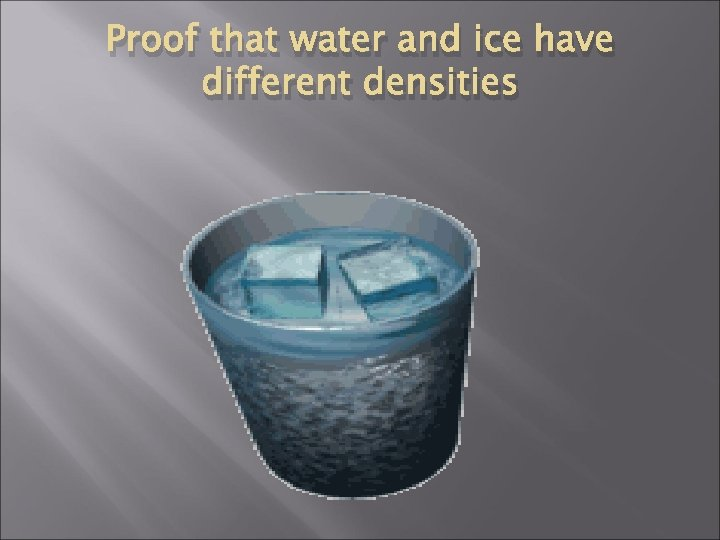Proof that water and ice have different densities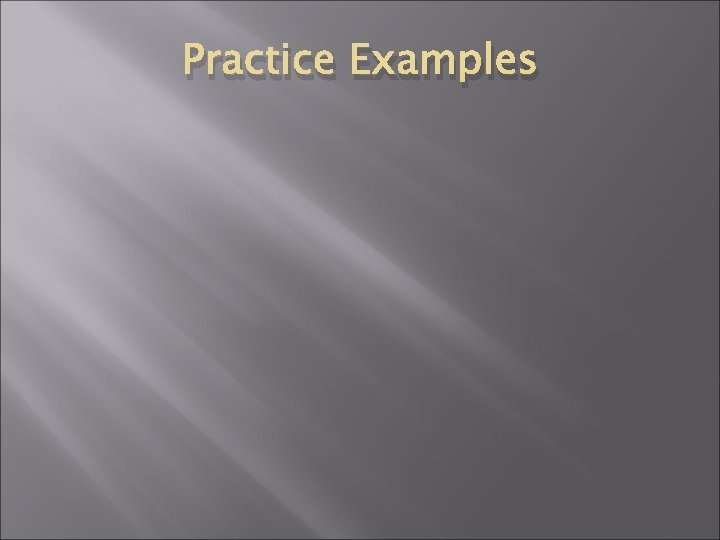Practice ExamplesTo find density: 1) Find the mass of the object 2) Find the volume of the object 3) Divide : Density = Mass - Volume Ex. If the mass of an object is 35 grams and it takes up 7 cm 3 of space, calculate the density.Let’s try a density problem together � Frank has a paper clip. It has a mass of 9 g and a volume of 3 cm 3. What is its density? � Frank also has an eraser. It has a mass of 3 g, and a volume of 1 cm 3. What is its density?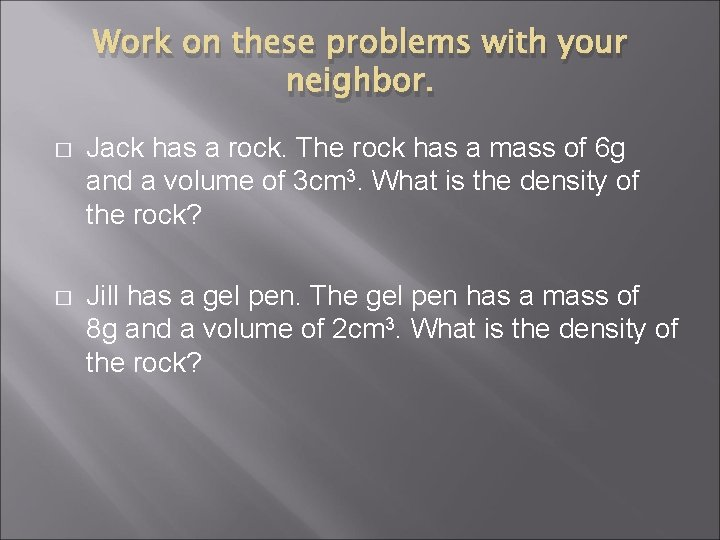Work on these problems with your neighbor. � Jack has a rock. The rock has a mass of 6 g and a volume of 3 cm 3. What is the density of the rock? � Jill has a gel pen. The gel pen has a mass of 8 g and a volume of 2 cm 3. What is the density of the rock?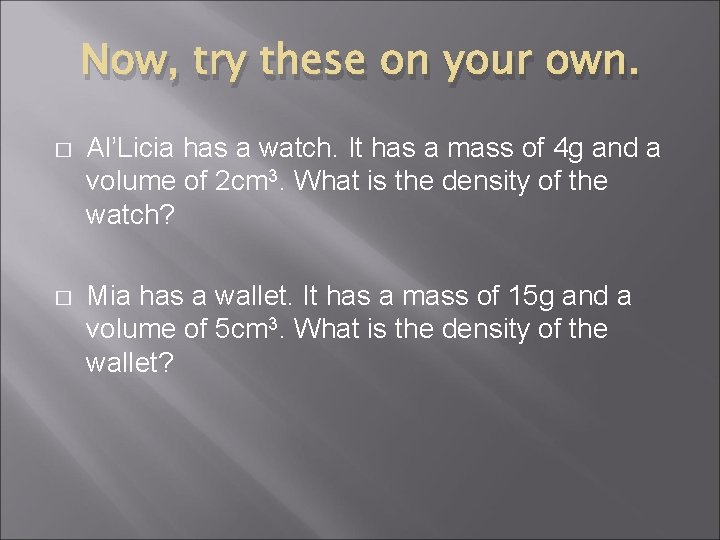Now, try these on your own. � Al’Licia has a watch. It has a mass of 4 g and a volume of 2 cm 3. What is the density of the watch? � Mia has a wallet. It has a mass of 15 g and a volume of 5 cm 3. What is the density of the wallet?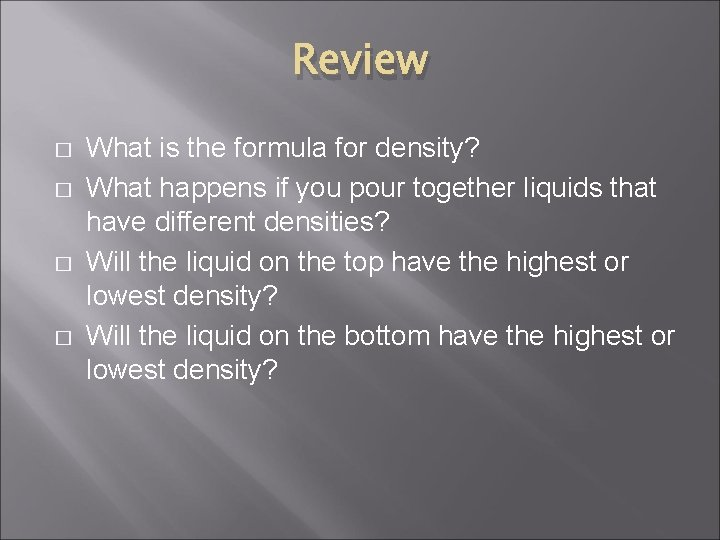Review � � What is the formula for density? What happens if you pour together liquids that have different densities? Will the liquid on the top have the highest or lowest density? Will the liquid on the bottom have the highest or lowest density?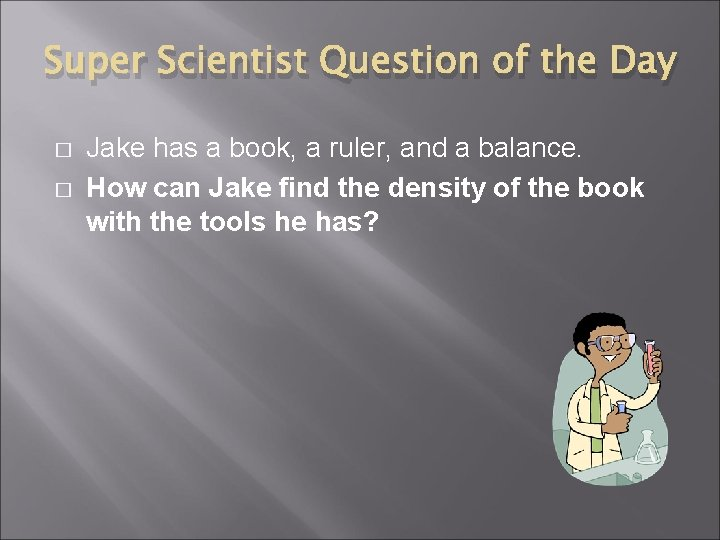Super Scientist Question of the Day � � Jake has a book, a ruler, and a balance. How can Jake find the density of the book with the tools he has?